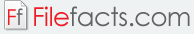### Derive 6

#### Texas Instruments Incorporated (Proprietary)

Derive 6 was a computer algebra system that was first released in 1988. It can be used for solving mathematical (algebra, calculus, trigonometry, and arithmetic) problems. Users can input problems with the mathematical symbols and Greek symbols that are built-in to the program. The application solves the problem and shows users the steps on how the final answer was derived. Plots of the problems can be done in 2D or 3D. Other features of the program for each mathematical area are listed below.

• Algebra
1. Solve systems in linear or non-linear
2. Define functions and assign variable values
3. Simplify expressions using powerful identities
• Arithmetic
1. Rational, scientific, and decimal notation
2. Support for real, imaginary, infinite, and complex numbers
3. Support for factoring large integers
• Calculus
1. Displays all the steps for simplification of expressions
2. Approximate numerical integration
3. Finite and infinite sums and products
• Vector, Sets, and Matrices
1. Support for symbolic and numeric elements
2. Provides functions for vector and matrix operations
3. Uses standard subscript notation
• Programming
1. Expression type recognizer functions
2. Extract and replace elements of vectors
3. Define default initial values for variables

Derive 6 comes with an extensive reference guide. More help topics can be found on the program’s website. The program was discontinued in 2007 and was replaces with the TI-Nspire graphing calculator.